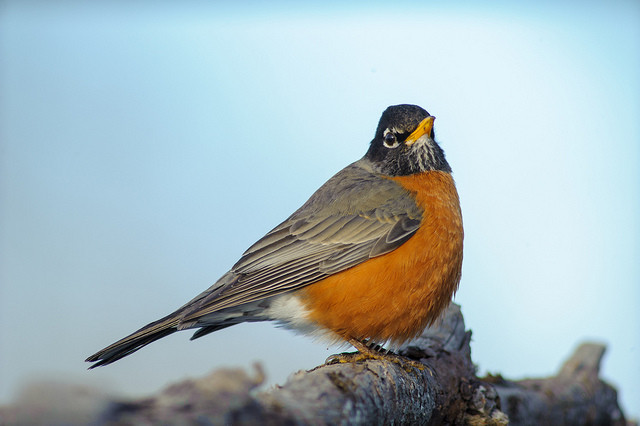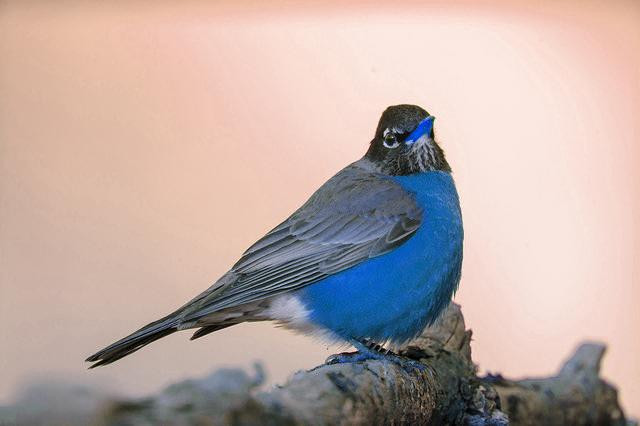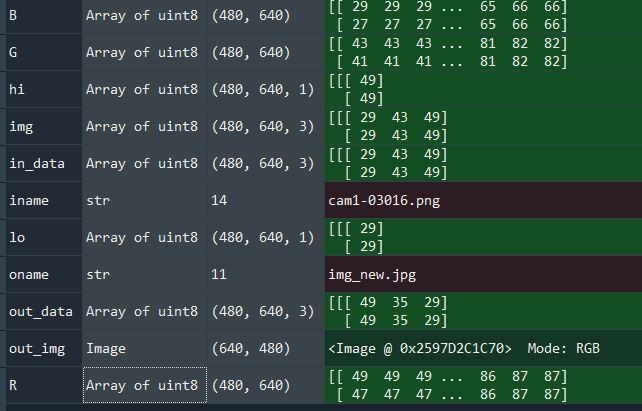#0

## 如何讓圖片變成互補色

tp655998 iT邦新手 5 級 ‧ 2021-04-09 00:55:37 檢舉

tp655998 iT邦新手 5 級 ‧ 2021-04-09 01:09:03 檢舉

https://www.includehelp.com/python/find-complementary-image-of-the-rgb-image.aspx

RGB xor FFFFFF
ARGB xor FFFFFFFF

http://www.vcskicks.com/image-invert.php

### 1 個回答

2

1. 白色RGB：(255, 255, 255)
原圖片+互補圖片=255
2. 黑色RGB：(0, 0, 0)
原圖片+互補圖片=256
3. 灰階RGB：255
原圖片+互補圖片=255

https://www.coder.work/article/1253704

``````    # 對每個像素每個顏色通道計算最大值/最小值
lo = np.amin(in_data, axis=2, keepdims=True)
hi = np.amax(in_data, axis=2, keepdims=True)
out_data = (lo + hi) - in_data
``````

``````import numpy as np
from PIL import Image

def complement_image(iname, oname):
img = Image.open(iname)
#img.show()

in_data = np.asarray(img)
#print(in_data.shape)

# 對每個像素每個顏色通道計算最大值/最小值
print('Complementing...')
lo = np.amin(in_data, axis=2, keepdims=True)
hi = np.amax(in_data, axis=2, keepdims=True)
out_data = (lo + hi) - in_data

out_img = Image.fromarray(out_data)
#out_img.show()
out_img.save(oname)
print('Saved to', oname)

complement_image('./bird.jpg', './bird_complement.jpg')
from IPython.display import Image
Image('./bird_complement.jpg', width=500)
``````tp655998 iT邦新手 5 級 ‧ 2021-04-09 19:22:34 檢舉

axis=2 是指圖片的RGB通道嗎

np.amin(in_data, axis=2, keepdims=True)

tp655998 iT邦新手 5 級 ‧ 2021-04-09 20:43:18 檢舉

amin的計算看的出來是圖片每個pixel的位置他rgb裡面取min值（過程很難用打字表達，所以省略）

tp655998 iT邦新手 5 級 ‧ 2021-04-10 16:15:05 檢舉